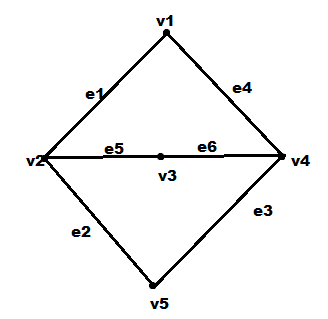QuestionAnswers

# What are the dimensions of an incidence matrix?A.Number of edges * number of edgesB.Number of edges * number of verticesC.Number of vertices * number of verticesD.None of the mentioned statements.

Hint: In an incidence matrix , the rows represent the vertices and the columns represent the edges.To fill in the incidence matrix , we need look at the name of the vertice in the row and the name of the edge in the column . If a vertex is connected by an edge we write 1 in the corresponding place .

Step 1:
A vertex is said to be incident to an edge if the edge is connected to the vertex
Let's look at an example
The graph below has five vertices and six edgesIn an incidence matrix , the rows represent the vertices and the columns represent the edges.
To fill in the incidence matrix , we need to look at the name of the vertice in the row and the name of the edge in the column . If a vertex is connected by an edge we write 1 in the corresponding place .
We can see that the edge ${e_1}$is incident with vertice ${v_1}$. So we write 1 in the corresponding place.
Same way we can fill all the places.
$\begin{gathered} \begin{array}{*{20}{c}} {}&{{\text{ }}{e_1}}&{{e_2}}&{{e_3}{\text{ }}{e_4}} \end{array}{\text{ }}{e_5}{\text{ }}{e_6} \\ \begin{array}{*{20}{c}} {{{\text{v}}_{\text{1}}}} \\ {{{\text{v}}_{\text{2}}}} \\ {{{\text{v}}_{\text{3}}}} \\ \begin{gathered} {{\text{v}}_{\text{4}}} \\ {{\text{v}}_{\text{5}}} \\ \end{gathered} \end{array}{\text{ }}\left[ \begin{gathered} \begin{array}{*{20}{c}} {\text{1}}&{\text{0}}&{\text{0}}&{\text{1}} \\ {\text{1}}&{\text{1}}&{\text{0}}&{\text{0}} \\ {\text{0}}&{\text{0}}&{\text{0}}&{\text{0}} \\ {\text{0}}&{\text{0}}&{\text{1}}&{\text{1}} \end{array}{\text{ }}\begin{array}{*{20}{c}} {\text{0}} \\ {\text{1}} \\ {\text{1}} \\ {\text{0}} \end{array}{\text{ }}\begin{array}{*{20}{c}} {\text{0}} \\ {\text{0}} \\ {\text{1}} \\ {\text{1}} \end{array} \\ \begin{array}{*{20}{c}} {\text{0}}&{\text{1}}&{{\text{ 1}}}&{\text{0}} \end{array}{\text{ }}\begin{array}{*{20}{c}} {\text{0}}&{\text{0}} \end{array} \\ \end{gathered} \right]{\text{ }} \\ \end{gathered}$
From this we can see that the dimensions of the incidence matrix is given by number of edges * number of vertices

The correct option is B.

Note:
The determinant of the incidence matrix of a closed loop is zero.
The rank of incidence matrix of a connected graph is (n–1).